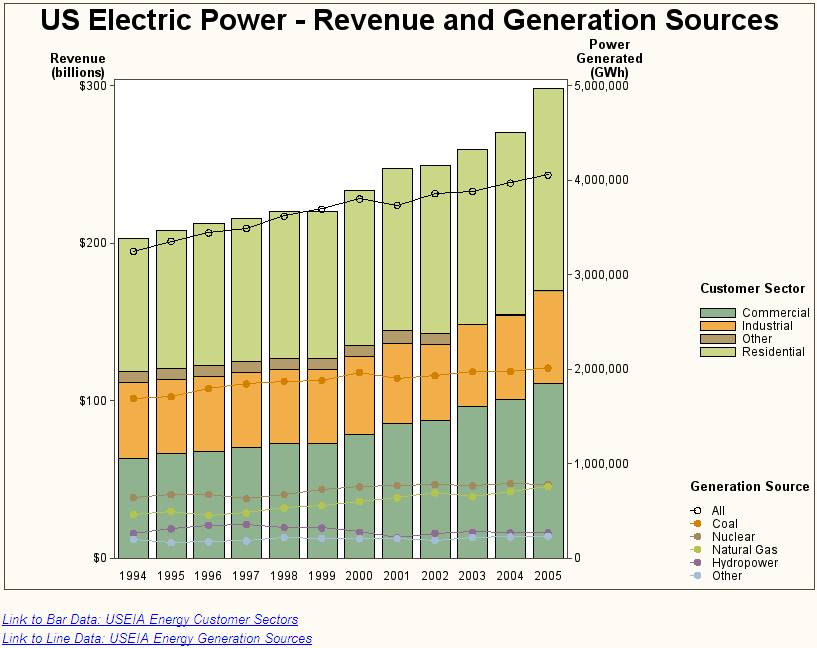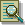The GBARLINE Procedure

Example 3: Specifying Subgroups, Multiple Plots, Data Tips, and Drill-Down URLs

Procedure Features:

BAR statement options:

 DISCRETE HTML= LEGEND= MAXIS= RAXIS= SUBGROUP= SUMVAR=

PLOT statement options:

 AXIS= HTML= LEGEND=

Multiple PLOT statements:

 SUMVAR=
Other Features:
 STYLE= option in the ODS statement AXIS statements LEGEND statements ODS HTML statement SYMBOL statement
Data set: SASHELP.ELECTRICThis graph shows the total amount of power generated by six different energy sources in the US during the years 1994 to 2005. It also shows the revenue received from four different customer sectors during these same years.

The power generated is graphed as a subgrouped bar chart. The chart variable is YEAR, and the subgroup variable is CUSTOMER, the customer sector. The program also specifies the DISCRETE option, so each year's data is graphed as a separate midpoint. The subgroups create a separate segment in the bar for each year, and the height of each bar represents the total revenue for that year for all customer sectors.

The power generated from each energy source is plotted as six different line plots. Each of the six plot lines represents a different energy source.

Separate legends are created for the bar chart and the line plots. By specifying the LEGEND POSITION= option, the legend for the bar chart is displayed at the top middle of the graph. The legend for the plots is displayed at the bottom right of the graph.

The colors used for everything except the plot lines is controlled by the style. The example specifies the Analysis style.

This example defines data tip text for both the plot symbols and the bar chart segments. It defines drill-down URLs for the entries in the footnotes.`goptions reset=all border;````ods listing close; ods html style=analysis gtitle nogfootnote;``````title1 "US Electric Power - Revenue and Generation Sources"; footnote1 j=r "GBLPOWER"; footnote2 j=l italic link="http://www.eia.doe.gov/cneaf/electricity/epa/epat7p3.html" "Link to Bar Data: USEIA Energy Customer Sectors"; footnote3 j=l italic link="http://www.eia.doe.gov/cneaf/electricity/epa/epat1p1.html" "Link to Line Data: USEIA Energy Generation Sources" ;``````axis1 label=(j=c "Revenue" j=c "(billions)") minor=none; /* left */ axis2 label=(j=c "Power" j=c "Generated" j=c "(GWh)") minor=none; /* right */ axis3 label=none; /* bottom */``````/* Bar legend */ legend1 position=(middle right outside) across=1 label=(position=(top ) j=l "Customer Sector"); /* Line plot legend */ legend2 position=(bottom right outside) across=1 repeat=1 label=(position=(top) j=l "Generation Source") ;``````symbol1 c=black value=circle; symbol2 value=dot;``````proc gbarline data=sashelp.electric; bar year / discrete sumvar=Revenue subgroup=Customer raxis=axis1 maxis=axis3 legend=legend1 html=revtip name="US_Electric_Power" des="Chart of US Electricity Generation Sources and Consumers"; plot / sumvar=AllPower html=alltip legend=legend2 axis=axis2; plot / sumvar=Coal html=coaltip; plot / sumvar=Nuclear html=nuketip; plot / sumvar=NaturalGas html=gastip; plot / sumvar=Hydro html=hydrotip; plot / sumvar=Other html=othertip; run; quit;``````ods HTML close; ods listing;```Previous Page | Next Page | Top of Page